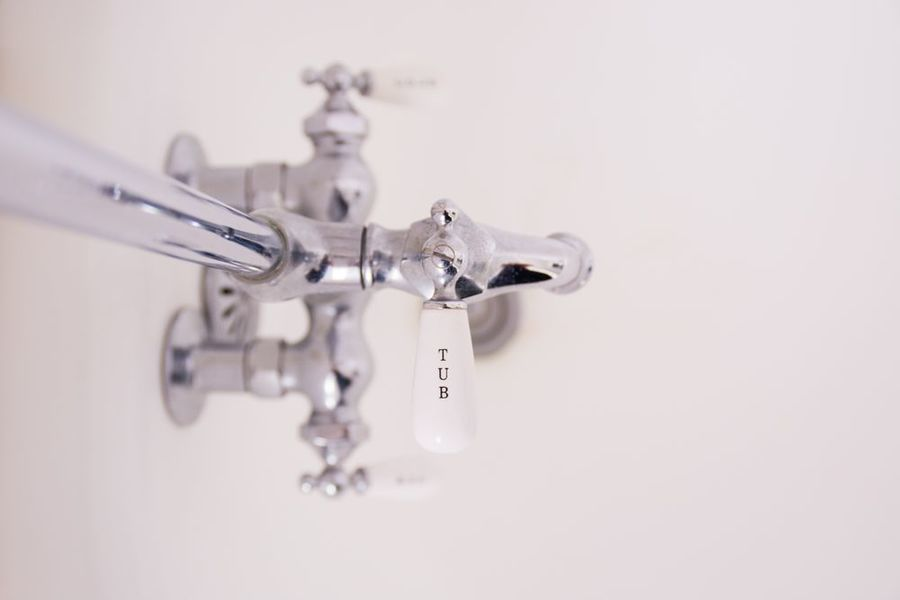#### How to fill a bath

By Dermot Tynan, over 3 years ago.

It seems straightforward enough to fill a bath. We know how to do it instinctively, but how do we program a computer to do it?

Let’s start with some assumptions. Assume we have a hot and cold tap, and we can control the flow on each tap. Also assume we can measure the average temperature of the water in the bath tub. Finally, assume we can measure the height of water in the bath. This last one is so we know when we’re done.

We’ll denote t as the measured temperature and l as the measured height of water. For that matter, we can refer to h as the amount of hot water, where 0.0 ≤ h ≤ 1.0 and c as the amount of cold water, again in the range 0.0 ≤ c ≤ 1.0.

Ultimately, we’re going to use a PID Controller to do the actual hard work of adjusting the taps. But before we get into that, we need to refine our equations just a bit, and also learn how to walk before we try to start running. (See what I did there? Running? Bath? Oh, never mind).

• April 3, 2018
• |
• |